D3D编程必备的数学知识（5） 转

平面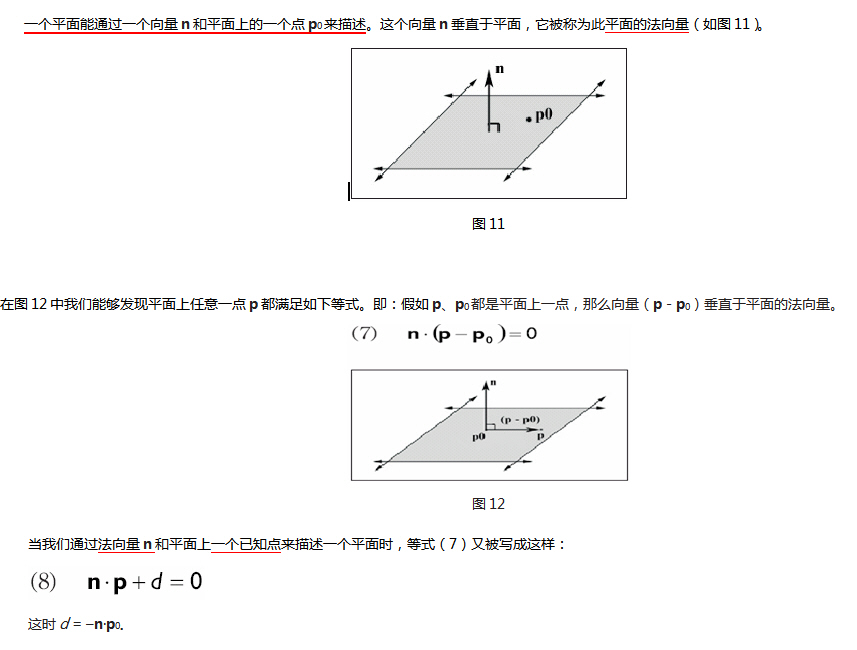D3DX平面

typedef struct D3DXPLANE
{
#ifdef __cplusplus
public:
D3DXPLANE() {}
D3DXPLANE( CONST FLOAT* );
D3DXPLANE( CONST D3DXFLOAT16* );
D3DXPLANE( FLOAT a, FLOAT b, FLOAT c, FLOAT d );

// casting
operator FLOAT* ();
operator CONST FLOAT* () const;

// unary operators
D3DXPLANE operator + () const;
D3DXPLANE operator - () const;

// binary operators
BOOL operator == ( CONST D3DXPLANE& ) const;
BOOL operator != ( CONST D3DXPLANE& ) const;
#endif //__cplusplus
FLOAT a, b, c, d;
} D3DXPLANE, *LPD3DXPLANE;

点和平面的空间关系

假如n·p + d >0，那么点p在平面的前面且在平面的正半空间里。

假如n·p + d <0，那么点p在平面的背面且在平面的负半空间里。

FLOAT D3DXPlaneDotCoord(

CONST D3DXPLANE *pP, // 平面

CONST D3DXVECTOR3 *pV // 点

);

// 测试点相对于平面的位置

D3DXPLANE p(0.0f, 1.0f, 0.0f, 0.0f);

D3DXVECTOR3 v(3.0f, 5.0f, 2.0f);

float x = D3DXPlaneDotCoord( &p, &v );

if( x approximately equals 0.0f ) // v在平面.上

if( x > 0 ) // v在正半空间

if( x < 0 ) // v在负半空间

创建平面

n·p0+ d = 0

n·p0 = -d

-n·p0 = d

D3DX库提供如下函数来完成创建平面的任务：

D3DXPLANE *D3DXPlaneFromPointNormal(

D3DXPLANE* pOut, // Result.

CONST D3DXVECTOR3* pPoint, // Point on the plane.

CONST D3DXVECTOR3* pNormal // The normal of the plane.

);

u = p1 - p0

v = p2 - p0

n = u × v

Then, -(n · p0) = d.

D3DX库提供如下函数来完成通过同一平面上的3个点确定一个平面：

D3DXPLANE *D3DXPlaneFromPoints(

D3DXPLANE* pOut, // Result.

CONST D3DXVECTOR3* pV1, // Point 1 on the plane.

CONST D3DXVECTOR3* pV2, // Point 2 on the plane.

CONST D3DXVECTOR3* pV3 // Point 3 on the plane.

);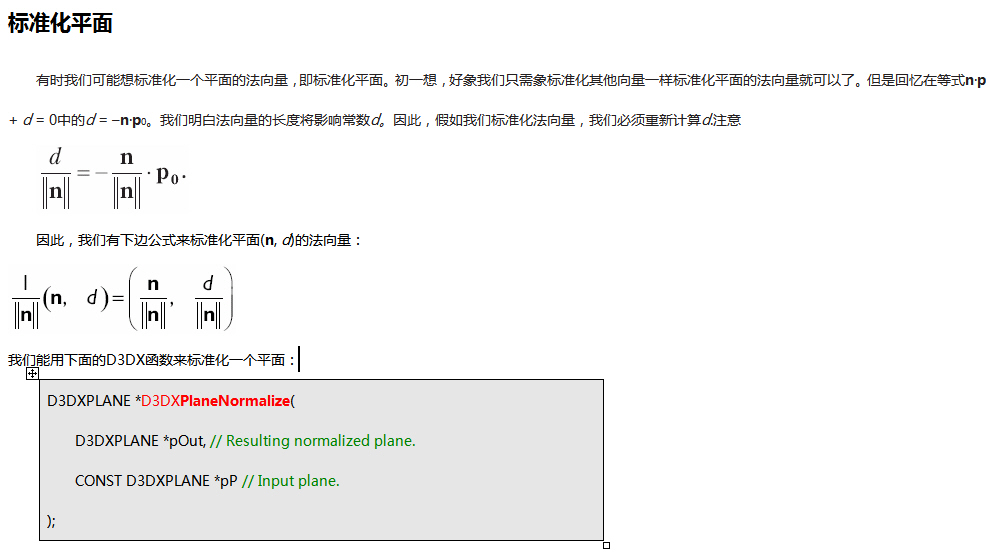变换平面

D3DXPLANE *D3DXPlaneTransform(

D3DXPLANE *pOut, // Result

CONST D3DXPLANE *pP, // Input plane.

CONST D3DXMATRIX *pM // Transformation matrix.

);

D3DXMATRIX T(...); // Init. T to a desired transformation.

D3DXMATRIX inverseOfT;

D3DXMATRIX inverseTransposeOfT;

D3DXMatrixInverse( &inverseOfT, 0, &T );

D3DXMatrixTranspose( &inverseTransposeOfT, &inverseOfT );

D3DXPLANE p(...); // Init. Plane.

D3DXPlaneNormalize( &p, &p ); // make sure normal is normalized.

D3DXPlaneTransform( &p, &p, &inverseTransposeOfT );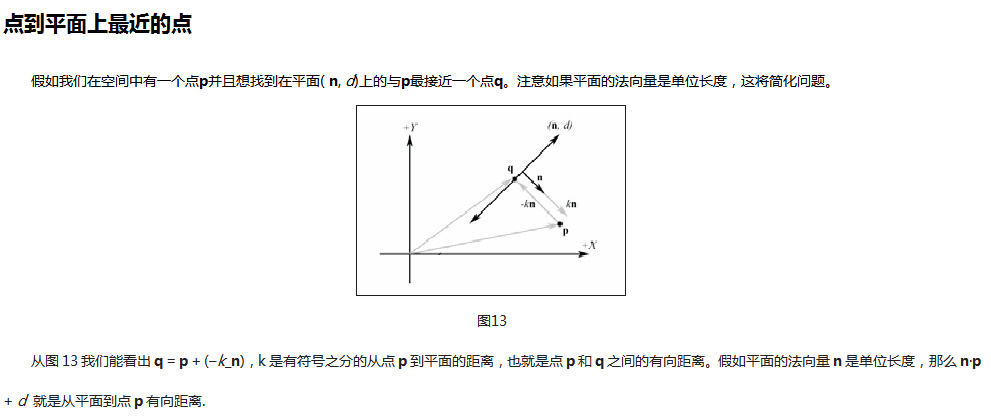射线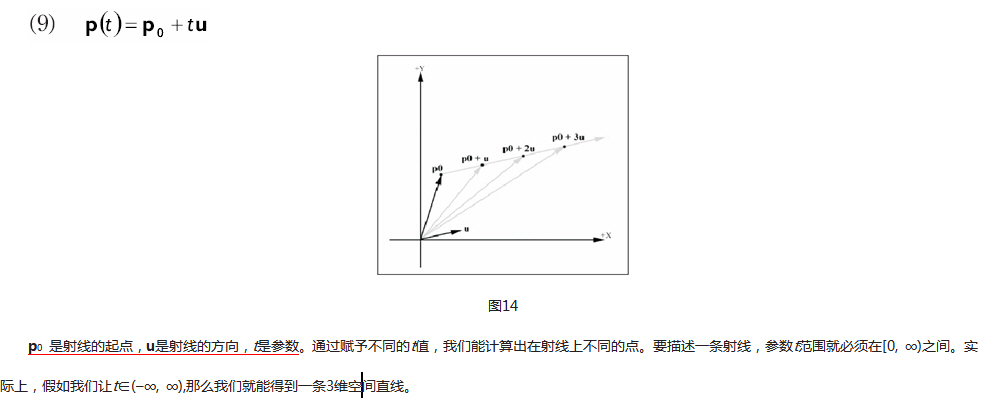线/面相交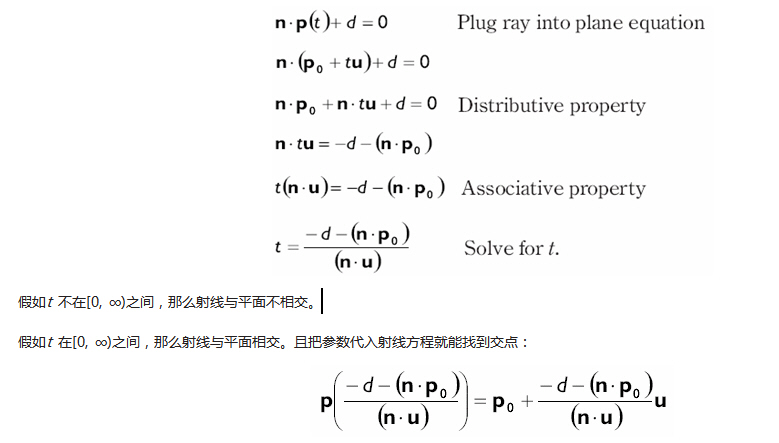rise-worlds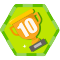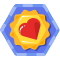yuer150
2011/04/26
375
3

yuer150
2011/05/02
1K
0

loving_forever_
2016/07/28
0
0

2018/11/08
0
0

W3Cschool
2018/04/18
0
0

8分钟前
0
0

11分钟前
1
0
Allegro非常实用的快捷键-PCB环境

demyar
18分钟前
0
0

19分钟前
0
0

tanghc
21分钟前
0
0